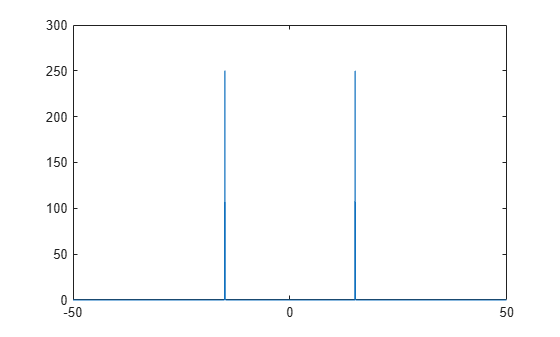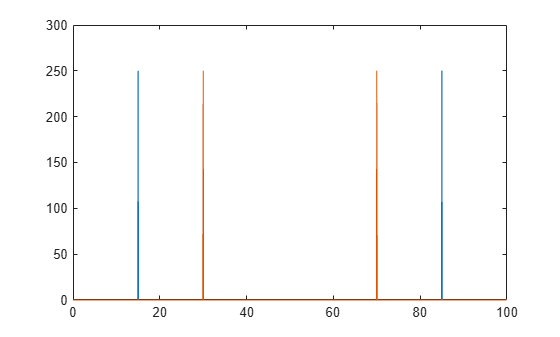# fftshift

Shift zero-frequency component to center of spectrum

## Syntax

``Y = fftshift(X)``
``Y = fftshift(X,dim)``

## Description

example

````Y = fftshift(X)` rearranges a Fourier transform `X` by shifting the zero-frequency component to the center of the array.If `X` is a vector, then `fftshift` swaps the left and right halves of `X`.If `X` is a matrix, then `fftshift` swaps the first quadrant of `X` with the third, and the second quadrant with the fourth.If `X` is a multidimensional array, then `fftshift` swaps half-spaces of `X` along each dimension.```

example

````Y = fftshift(X,dim)` operates along the dimension `dim` of `X`. For example, if `X` is a matrix whose rows represent multiple 1-D transforms, then `fftshift(X,2)` swaps the halves of each row of `X`.```

## Examples

collapse all

Swap the left and right halves of a row vector. If a vector has an odd number of elements, then the middle element is considered part of the left half of the vector.

```Xeven = [1 2 3 4 5 6]; fftshift(Xeven)```
```ans = 1×6 4 5 6 1 2 3 ```
```Xodd = [1 2 3 4 5 6 7]; fftshift(Xodd)```
```ans = 1×7 5 6 7 1 2 3 4 ```

When analyzing the frequency components of signals, it can be helpful to shift the zero-frequency components to the center.

Create a signal `S`, compute its Fourier transform, and plot the power.

```fs = 100; % sampling frequency t = 0:(1/fs):(10-1/fs); % time vector S = cos(2*pi*15*t); n = length(S); X = fft(S); f = (0:n-1)*(fs/n); %frequency range power = abs(X).^2/n; %power plot(f,power)```Shift the zero-frequency components and plot the zero-centered power.

```Y = fftshift(X); fshift = (-n/2:n/2-1)*(fs/n); % zero-centered frequency range powershift = abs(Y).^2/n; % zero-centered power plot(fshift,powershift)```You can process multiple 1-D signals by representing them as rows in a matrix. Then use the dimension argument to compute the Fourier transform and shift the zero-frequency components for each row.

Create a matrix `A` whose rows represent two 1-D signals, and compute the Fourier transform of each signal. Plot the power for each signal.

```fs = 100; % sampling frequency t = 0:(1/fs):(10-1/fs); % time vector S1 = cos(2*pi*15*t); S2 = cos(2*pi*30*t); n = length(S1); A = [S1; S2]; X = fft(A,[],2); f = (0:n-1)*(fs/n); % frequency range power = abs(X).^2/n; % power plot(f,power(1,:),f,power(2,:))```Shift the zero-frequency components, and plot the zero-centered power of each signal.

```Y = fftshift(X,2); fshift = (-n/2:n/2-1)*(fs/n); % zero-centered frequency range powershift = abs(Y).^2/n; % zero-centered power plot(fshift,powershift(1,:),fshift,powershift(2,:))```## Input Arguments

collapse all

Input array, specified as a vector, a matrix, or a multidimensional array.

Data Types: `double` | `single` | `int8` | `int16` | `int32` | `int64` | `uint8` | `uint16` | `uint32` | `uint64` | `logical`
Complex Number Support: Yes

Dimension to operate along, specified as a positive integer scalar. If no value is specified, then `fftshift` swaps along all dimensions.

• Consider an input matrix `Xc`. The operation `fftshift(Xc,1)` swaps halves of each column of `Xc`.• Consider a matrix `Xr`. The operation `fftshift(Xr,2)` swaps halves of each row of `Xr`.Data Types: `double` | `single` | `int8` | `int16` | `int32` | `int64` | `uint8` | `uint16` | `uint32` | `uint64` | `logical`